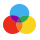Colors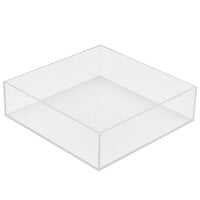$25.99/EachColors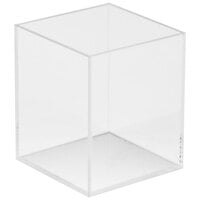$14.99/EachColors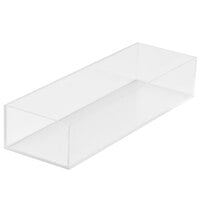$25.49/Each From$40.15/Each

Reg.Lots of 6
$42.99$40.15Colors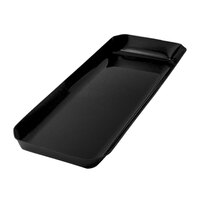From $56.75/Each Reg.Lots of 6$60.49$56.75Colors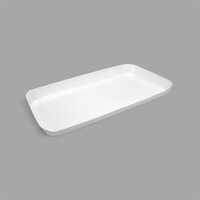From$56.75/Each

Reg.Lots of 6
$60.49$56.75Colors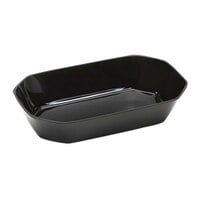From $21.71/Each Reg.Lots of 6$22.99$21.71Colors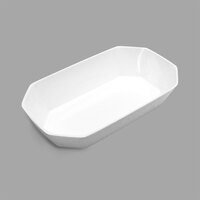From$21.71/Each

Reg.Lots of 6
$22.99$21.71Colors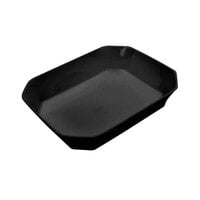From $23.26/Each Reg.Lots of 6$24.99$23.26Colors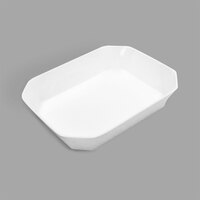From$23.26/Each

Reg.Lots of 6
$24.99$23.26Colors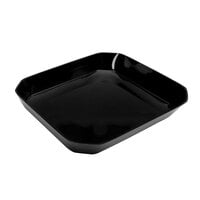From $26.74/Each Reg.Lots of 6$28.49$26.74Colors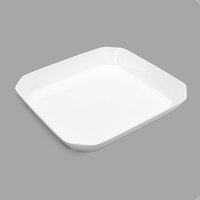From$26.74/Each

Reg.Lots of 6
$28.49$26.74Colors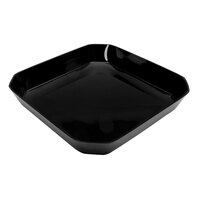From $31.36/Each Reg.Lots of 6$33.49$31.36Colors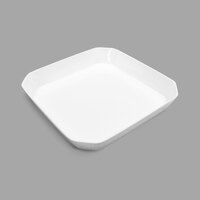From$31.36/Each

Reg.Lots of 6
$33.49$31.36Colors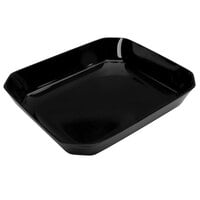From $29.16/Each Reg.Lots of 6$30.99$29.16Colors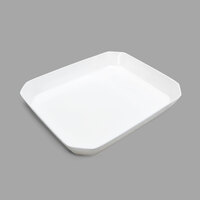From$29.16/Each

Reg.Lots of 6
$30.99$29.16Colors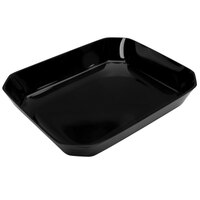From $38.69/Each Reg.Lots of 6$41.49$38.69Colors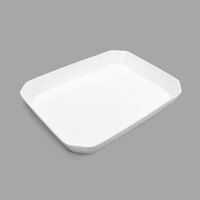From$38.69/Each

Reg.Lots of 6
$41.49$38.69Colors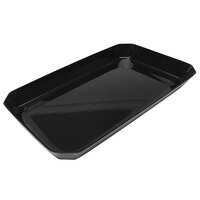From $40.15/Each Reg.Lots of 6$42.99$40.15Colors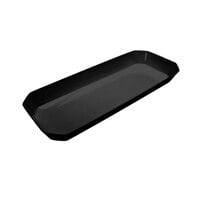From$31.07/Each

Reg.Lots of 6
$32.99$31.07Colors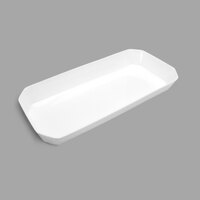From $31.07/Each Reg.Lots of 6$32.99$31.07Colors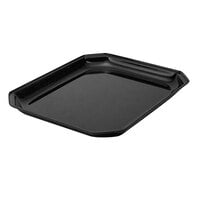From$20.71/Each

Reg.Lots of 6
$21.99$20.71Colors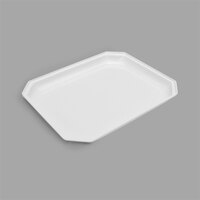From $20.71/Each Reg.Lots of 6$21.99$20.71Colors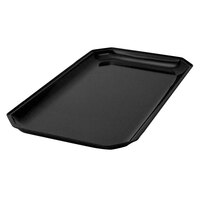From$22.53/Each

Reg.Lots of 6
$23.99$22.53Colors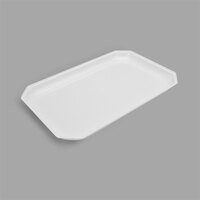From $22.53/Each Reg.Lots of 6$23.99$22.53Colors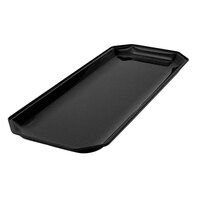From$23.59/Each

Reg.Lots of 6
$24.99$23.59Colors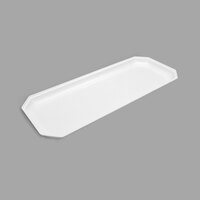From $23.59/Each Reg.Lots of 6$24.99\$23.59